# What’s so complex about complex algebra?

Title: Isolating Approaches: How middle-division physics students coordinate forms and representations in complex algebra
Authors: Emily M. Smith, Justyna P. Zwolak, and Corinne A. Manogue
First author institution: Oregon State University
Journal: Physical Review Physics Education Research 15, 010138 (2019)

Being able to change between representations is a key skill needed to solve physics problems. Think about how difficult it would be to solve force problems if you could only use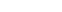or draw free body diagrams. Yet, while using multiple representations is often encouraged in introductory physics, the focus switches to more algebraic representations in upper-division courses such as quantum mechanics, despite the usefulness of graphical or geometric representations. Switching between these representations becomes especially useful in upper-division physics once complex numbers are introduced since some problems are easier to solve when using a specific representation.

Prior work has shown that students struggle with complex algebra in physics and multiple representations of complex numbers in general. For example, upper-division mathematics students tend to use algebraic rather than geometric representations to solve problems and even when prompted to use geometric reasoning, reason first algebraically and then shift to geometric reasoning. However, there have been no studies of physics students’ abilities with complex algebra without a physics context, which can add complexity (no pun intended) to the problem. The goal of this study is then to understand physics students’ difficulties and successful strategies for solving a straightforward complex algebra problem.

The authors first identified the possible ways a student could represent a complex number, resulting in six possible pairs, based on the representation (algebraic or geometric) and the type of coordinates used, which are all shown in figure 1.Figure 1: The various forms and representations for expressing a complex number. (Table 1 in paper).

Since some problems are solved more easily by using a specific representation, the authors created a straightforward problem which required the student to use a geometric representation to find the algebraic form in one of the various coordinate systems. The problem is shown in figure 2.Figure 2: The problem students were asked to solve. Part a is most easily solved using rectangular form first while part b is most easily solved using polar or exponential form first. (Figure 1 in paper).

To understand how students attempt to solve the problem, the researchers interviewed 7 junior physics majors at a large, public, research university during week 5 of a semester long physics course. The course had included an introduction to complex algebra during the first week and assessments of complex algebra during the first through third weeks of the course. However, none of the physics material required the use of complex algebra.

The students each worked through the problem with a researcher present, and verbalized their thought process to the researcher as they worked through the problem. The researcher did not provide the student with part b of the problem until they have completed part a.

After all 7 had worked with one of the researchers, the researchers classified parts of the written transcript of the student solving the problem based on the approach they took. For example, the student could have drawn a triangle and used the Pythagorean theorem to find the real side since the magnitude of the complex number is the length of the hypotenuse of the triangle or could have used sine and cosine along with the magnitude of the complex number to find the real and imaginary parts; these approaches were referred to as triangular approaches and are shown in figure 3.Figure 3: Possible ways to represent z in complex form using either triangle trigonometry (left) or the Pythagorean theorem (right). (Based on figure 1 in the paper).

Alternatively, the student could have used a circular or polar representation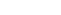to find the real and imaginary parts. In this case, the student would have needed to know that the angle used here is measured counter-clockwise from the positive, real axis and not just the angle shown in the figure. This approach, called the circular approach, is shown in figure 4.Figure 4: Using the circular approach to solve the problem. The student needs to notice that the polar angle is and not just α. (Based on figure 1 in paper).

When looking at student responses, the researchers found that none of the students took a direct approach to solve the problem and instead most took steps that didn’t fit within a single approach. For example, one of the students started by calculating the norm of the complex number (an approach that doesn’t fit within either the triangular or circular approach), finding that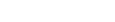. He then pulls from his knowledge that the real part is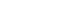and the imaginary part is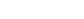, but does not replace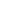with the equation he found earlier. Instead, he appears to abandon that line of reasoning. This broad pattern of starting an approach before discarding it was used by 4 of the 7 students.

Across all seven students, the researchers found that students who mixed the approaches also tended to make mistakes in combining those approaches. For example, one student found the real and imaginary part correctly using the Pythagorean theorem but then multiplied the results by cos(α) and sin(α) respectively as one would do if they were using a triangular trigonometry approach, which is not necessary if using the Pythagorean theorem.

Alternatively, some students blended the triangular and circular approaches. For example, one student used the correct formula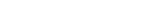(circular approach) but then incorrectly used the angle of the triangle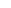as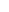(triangular approach). Further, the student recognized that the imaginary component should be negative but rather than seeing that the angle should account for this, the student inserted a negative sign in front of the imaginary part, incorrectly resulting in a negative real part as well. While the student realized he needed to get a positive value for the real part, he was unable to make the algebra match his intuition.

Finally, four of the students consistently worked in rectangular form until they needed to switch to exponential form to solve the problem. As part b is actually easier to solve using the exponential form first, the authors suggest this shows the students have a preference for rectangular form. While the authors acknowledge that the wording of the question may have contributed to which coordinates students used first, the authors note that none of the advanced undergraduate students or graduate students who piloted the question showed this preference.

While the study only observed seven students solving the problem, there are some teaching implications of the work. First, students have difficulties transitioning between geometric and algebraic representations of complex numbers. This was even after the student’s course included some discussion of complex numbers; however, the discussion occurred a few weeks before the study. Second, students who used a single approach were more successful in solving the problem than students who mixed approaches. Finally, the biggest difficulty for the students was distinguishing between triangular and circular trigonometry, with students often mixing up which angle to use. Instructors could address this when introducing polar coordinates and include examples outside of the first quadrant where the polar and triangle angles are not the same.